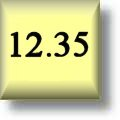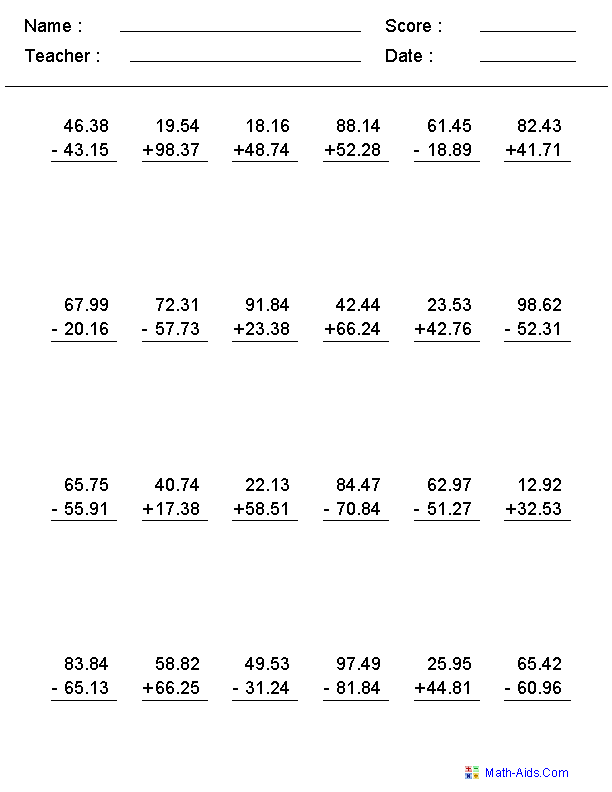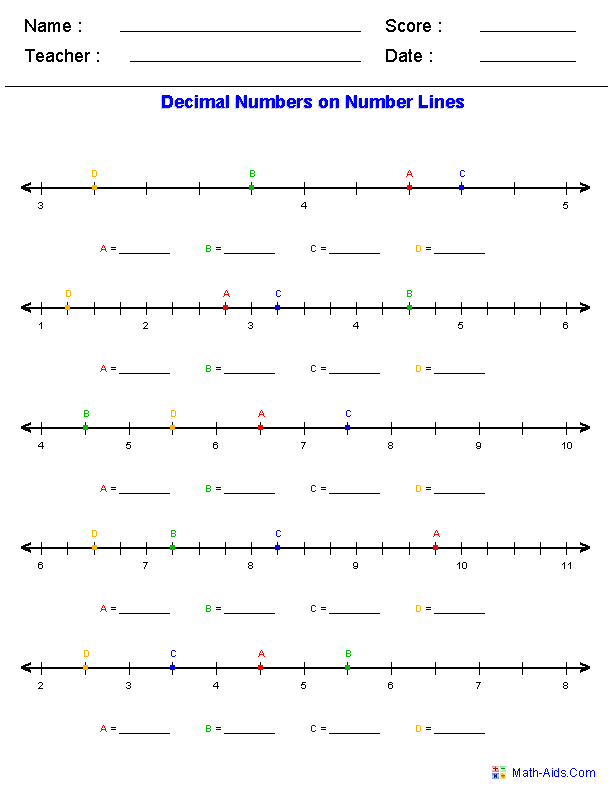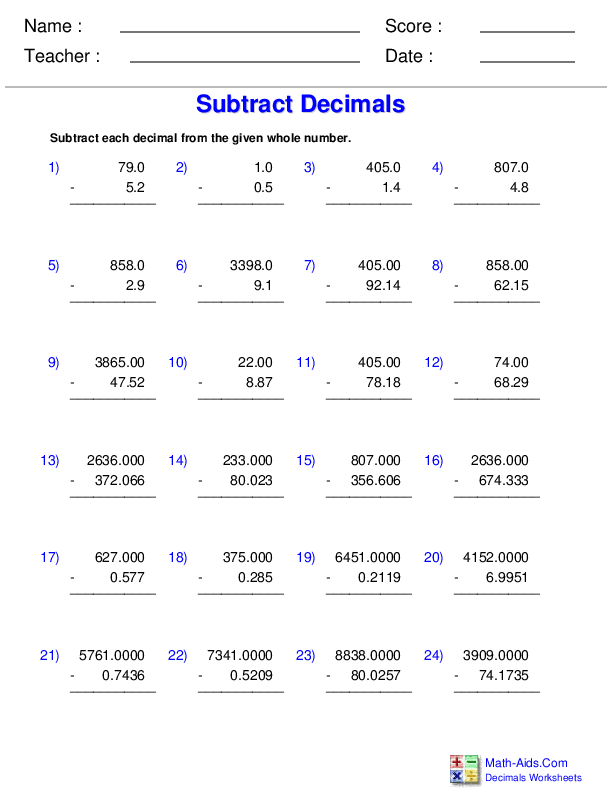#Decimals Worksheets

## Dynamically Created Decimal Worksheets

Here is a graphic preview for all of the Decimals Worksheets. You can select different variables to customize these Decimals Worksheets for your needs. The Decimals Worksheets are randomly created and will never repeat so you have an endless supply of quality Decimals Worksheets to use in the classroom or at home. Our Decimals Worksheets are free to download, easy to use, and very flexible.

These Decimals Worksheets are a great resource for children in Kindergarten, 1st Grade, 2nd Grade, 3rd Grade, 4th Grade, and 5th Grade.

Click here for a Detailed Description of all the Decimals Worksheets.

## Quick Link for All Decimals Worksheets

Click the image to be taken to that Decimals Worksheet.

##### Addition WorksheetsWith Decimals##### Subtraction WorksheetsWith Decimals##### Multiplication WorksheetsWith Decimals##### Add & Subtract WorksheetsWith Decimals##### Decimal Long Division Decimal Worksheets##### 3 Decimal Division Decimal Worksheets##### Mixed Quotient Division Decimal Worksheets##### Rounding WorksheetsWith Decimals##### Comparing WorksheetsWith Decimals##### Number Lines WorksheetsWith Decimals##### Multiplying by Powers of TenWith Decimals##### Ordering NumbersWith Decimals##### Subtract Decimals from Whole NumbersRecommended Videos

## Detailed Description for All Decimals Worksheets

Addition Worksheets with Decimals
These decimals worksheets may be configured for 1, 2, or 3 digits on the right of the decimal and up to 4 digits on the left of the decimal as well as 2, 3, and 4 addends addition problems for these decimals worksheets.

Subtraction Worksheets with Decimals
These decimals worksheets may be configured for 1, 2, and 3 Digits on the right of the decimal and up to 4 digits on the left of the decimal subtraction problems. You may select up to 25 subtraction problems for these decimals worksheets.

Multiplication Worksheets with Decimals
These decimals worksheets may be configured for 1 or 2 Digits on the right of the decimal and up to 2 digits on the left of the decimal. You may vary the numbers of multiplication problems on the decimals worksheets from 12 to 25.

Add & Subtract Worksheets with Decimals
These decimals worksheets may be configured for 1, 2, and 3 Digits on the right of the decimal and up to 4 digits on the left of the decimal. You may select up to 25 addition and subtraction problems per worksheet.

Decimal Long Division Worksheets
These decimal worksheets allows for the number of digits in the divisors to be varied from 1 to 3. You may select the number of decimals in the dividend for the problems. These decimal worksheets produce 9 problems per worksheet.

3 Digit Decimal Division Worksheets
Horizontal Format

These decimal worksheets produces problems in which you must divide a 3 digit decimal number by a single digit number. You may select between 12, 15, 18, 21, 24 or 30 problems for these decimal worksheets.

Mixed Quotient Division Worksheets
These decimal worksheets will produce problems with mixed formats for the quotient, but keeping the divisor and dividend as whole numbers. You may select either whole numbers, one decimal, two decimals, or a mixture of all types of problems. The decimal worksheet will produce 9 problems per worksheet.

Rounding Worksheets with Decimals
These decimals worksheets are great for teaching children to round decimal numbers to the nearest tenths, hundredths, or thousandths.

Comparing Decimal Numbers Worksheets
These decimal worksheets are great for testing children in their comparison of pairs of decimal numbers. You may select the problems to be positive, negative, or mixed.

Number Lines Worksheets with Decimals
These decimals worksheets will produce problems for the children to correctly mark the mixed numbers on the given number lines. You may select positive or negative decimals for the problems.

Multiplying by Powers of Ten with Decimals
These decimals worksheets will produce decimal multiplication problems with facts being powers of ten. It may be configured for 1, 2, or 3 digits on the right of the decimal and up to 2 digits on the left. You may vary the numbers of problems on each worksheet from 12 to 25.

Ordering Decimal Numbers Worksheets
These decimals worksheets will produce problems that involve ordering decimal numbers. The student will be given a list of decimal numbers and be asked to order them in ascending or descending order. You may select the number of problems per worksheets, the number of decimal numbers to sort per problem, the number of digits in each decimal number, as well as the way to order the decimal numbers.

Subtract Decimals from Whole Numbers Worksheets
These decimals worksheets will produce problems that ask students to subtract decimals from whole numbers. The student will be given subtraction problems of decimals and whole numbers and be asked to solve them. You may select the number ranges, whether to only have positive answers, and up to 24 problems per worksheet.